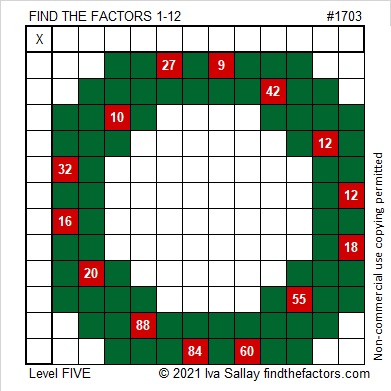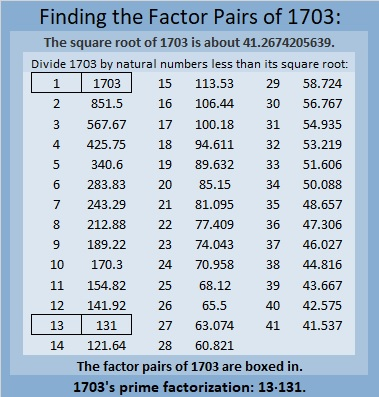# 1703 A Wreath to Hang on Your Door

Contents

### Today’s Puzzle:

A wreath is a lovely decoration to hang on your door at Christmastime. This one might have a few thorns in it, but if you are careful, they won’t bother you in the least. Just use logic to write the numbers 1 to 12 in both the first column and the top row so that those numbers and the given clues create a multiplication table.### Factors of 1703:

• 1703 is a composite number.
• Prime factorization: 1703 = 13 × 131.
• 1703 has no exponents greater than 1 in its prime factorization, so √1703 cannot be simplified.
• The exponents in the prime factorization are 1 and 1. Adding one to each exponent and multiplying we get (1 + 1)(1 + 1) = 2 × 2 = 4. Therefore 1703 has exactly 4 factors.
• The factors of 1703 are outlined with their factor pair partners in the graphic below.### More About the Number 1703:

Did you notice a cool pattern in 1703’s prime factorization?
13·131 =1703.

1703 is the hypotenuse of a Pythagorean triple:
655-1572-1703, which is (5-12-13) times 131.

1703 is the difference of two squares in two different ways:
852² – 851² = 1703, and
72² – 59² = 1703.

This site uses Akismet to reduce spam. Learn how your comment data is processed.